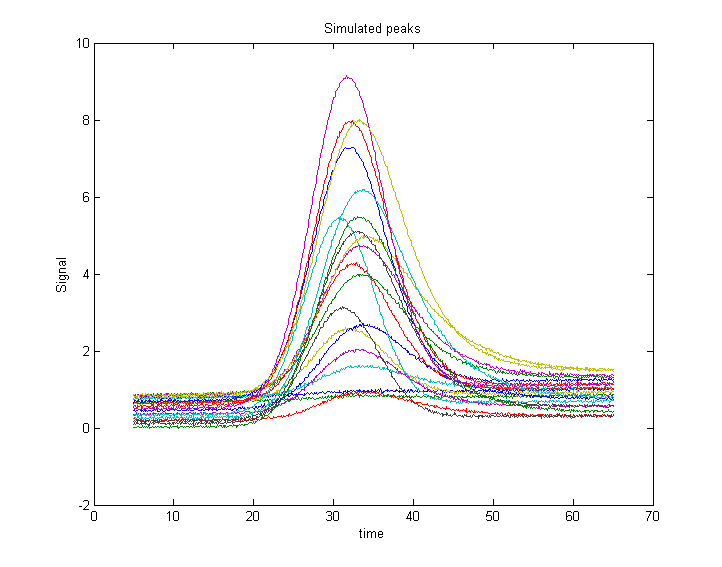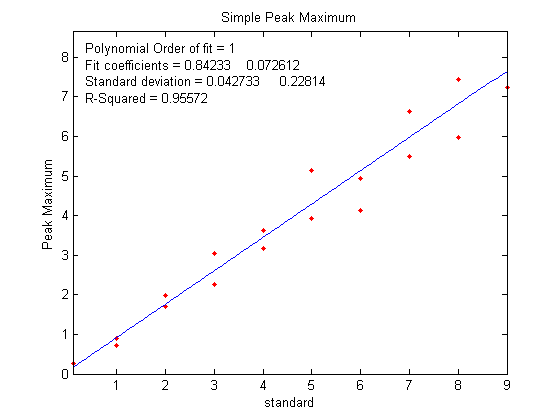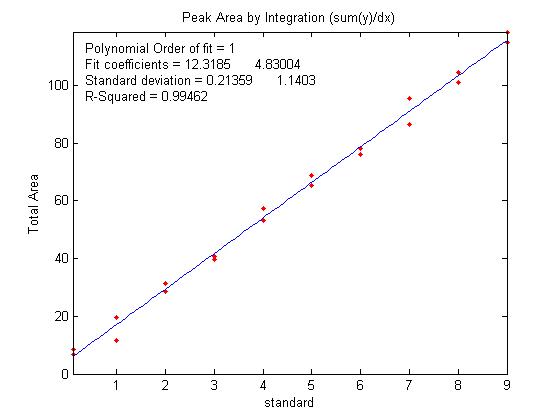[Introduction]  [Signal arithmetic]  [Signals and noise]   [Smoothing]   [Differentiation]  [Peak Sharpening]  [Harmonic analysis]   [Fourier convolution]  [Fourier deconvolution]  [Fourier filter]  [Wavelets]   [Peak area measurement]  [Linear Least Squares]  [Multicomponent Spectroscopy]  [Iterative Curve Fitting]  [Hyperlinear quantitative absorption spectrophotometry] [Appendix and Case Studies]  [Peak Finding and Measurement]  [iPeak]   [iSignal]  [Peak Fitters]   [iFilter]  [iPower]  [List of downloadable software]  [Interactive tools]

### AppendixL: Why measure peak area rather than peak height?

This appendix examines more closely the question of measuring peak area rather than peak height to reduce the effect of peak broadening, which commonly occurs in chromatography, for reasons that are discussed previously, and also in some forms of spectroscopy. Under what conditions the measurement of peak area might be better than peak height?

The Matlab/Octave script "HeightVsArea.m" simulates the measurement of a series of standard samples whose concentrations are given by the vector 'standards'. Each standard produces an isolated peak whose peak height is directly proportional to the corresponding value in 'standards' and whose underlying shape is a Gaussian with a constant peak position ('pos') and width ('wid'). To simulate the measurement of these samples under typical conditions, the script changes the shape of the peaks (by exponential broadening) and adds a variable baseline and random noise. You can control, by means of the variable definitions in the first few lines of the script, the peak beginning and end, the sampling rate 'deltaX' (increment between x values), the peak position and width ('pos' and 'wid'), the sequence of peak heights ('standards'), the baseline amplitude ('baseline') and its degree of variability ('vba'), the extent of shape change ('vbr'), and the amount of random noise added to the final signal ('noise').

The resulting peaks are shown in Figure 1. The script prepares a series of "calibration curves" plotting the values of 'standard' against the measured peak heights or areas for each measurement method. The measurement methods include peak height in Figure 2, peak area in Figure 3, and curve fitting height and area in Figures 4 and 5, respectively. These plots should ideally have an intercept of zero and an R2 of 1.000, but the slope is greater for the peak area measurements because area has different units and is numerically greater than peak height. All the measurement methods are baseline corrected; that is, they include code that attempts to compensate for changes in the baseline (controlled by the variable 'baseline').

With the initial values of 'baseline', 'noise', 'vba', and 'vbr', you can clearly see the advantage of peak area measurements (figure 3) compared to peak height (figure 2). This is primarily due to the effect of the variability of peak shape broadening ('vbr') and to the averaging out of random noise in the computation of area.Figure 2                                                                           Figure 3

If you set 'baseline', 'noise', 'vba', and 'vbr' all to zero, you've simulated a perfect world in which all methods work perfectly.

Curve fitting can measure both peak height and area; it is not even absolutely necessary to use an accurate peak shape model. Using a simple Gaussian model in this example works much better for peak area (Figure 5) than for peak height (Figure 4) but is not significantly better than a simple peak area measurement (Figure 3). The best results are obtained if an exponentially-broadened Gaussian model (shape 31 or  39) is used, using the code in line 30, but that computation takes longer. Moreover, if the measured peak overlaps another peak significantly, curve fitting both of those peaks together can give much more accurate results that other peak area measurement methods.

This page is part of "A Pragmatic Introduction to Signal Processing", created and maintained by Prof. Tom O'Haver , Department of Chemistry and Biochemistry, The University of Maryland at College Park. Comments, suggestions and questions should be directed to Prof. O'Haver at toh@umd.edu. Updated July, 2022.